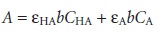Home | | Modern Analytical Chemistry | Limitations to Beer’s Law

# Limitations to Beer’s Law

According to Beer’s law, a calibration curve of absorbance versus the concentration of analyte in a series of standard solutions should be a straight line with an intercept of 0 and a slope of ab or εb.

Limitations to Beer’s Law

According to Beer’s law, a calibration curve of absorbance versus the concentration of analyte in a series of standard solutions should be a straight line with an intercept of 0 and a slope of ab or εb. In many cases, however, calibration curves are found to be nonlinear (Figure 10.22). Deviations from linearity are divided into three cate- gories: fundamental, chemical, and instrumental.## Fundamental Limitations to Beers Law

Beer’s law is a limiting law that is valid only for low concentrations of analyte. There are two contributions to this funda- mental limitation to Beer’s law. At higher concentrations the individual particles of analyte no longer behave independently of one another. The resulting interaction between particles of analyte may change the value of ε. A second contribution is that the absorptivity, a, and molar absorptivity, ε, depend on the sample’s refractive index. Since the refractive index varies with the analyte’s concentration, the values of a and ε will change. For sufficiently low concentrations of analyte, the refractive index remains essentially constant, and the calibration curve is linear.

## ChemicalLimitationsto Beer’s Law

Chemical deviations from Beer’s law can occur when the absorbing species is involved in an equilibrium reaction. Consider, as an example, an analysis for the weak acid, HA. To construct a Beer’s law calibra- tion curve, several standards containing known total concentrations of HA, Ctot, are prepared and the absorbance of each is measured at the same wavelength. Since HA is a weak acid, it exists in equilibrium with its conjugate weak base, AIf both HA and Aabsorb at the selected wavelength, then Beers law is written as10.7

where CHA and CA are the equilibrium concentrations of HA and A. Since the weak acid’s total concentration, Ctot, isthe concentrations of HA and Acan be written as

CHA = αHACtot        …… 10.8

CA = (1– αHA)Ctot        …… 10.9

where αHA is the fraction of weak acid present as HA. Substituting equations 10.8 and 10.9 into equation 10.7, and rearranging, gives

A = HAαHA + εA εAαHA)bCtot        …… 10.10

Because values of αHA may depend on the concentration of HA, equation 10.10 may not be linear. A Beer’s law calibration curve of A versus Ctot will be linear if one of two conditions is met. If the wavelength is chosen such that εHA and εA are equal, then equation 10.10 simplifies to

A = εAbCtot

and a linear Beer’s law calibration curve is realized. Alternatively, if αHA is held con- stant for all standards, then equation 10.10 will be a straight line at all wavelengths. Because HA is a weak acid, values of αHA change with pH. To maintain a constant value for αHA, therefore, we need to buffer each standard solution to the same pH. Depending on the relative values of εHA and εA, the calibration curve will show a positive or negative deviation from Beer’s law if the standards are not buffered to the same pH.

## Instrumental Limitations to Beer’s Law

There are two principal instrumental limi- tations to Beer’s law. The first limitation is that Beer’s law is strictly valid for purely monochromatic radiation; that is, for radiation consisting of only one wavelength. As we learned, however, even the best wavelength selector passes radiation with a small, but finite effective bandwidth. Using polychromatic radia- tion always gives a negative deviation from Beer’s law, but is minimized if the value of ε is essentially constant over the wavelength range passed by the wavelength se- lector. For this reason, as shown in Figure 10.23, it is preferable to make absorbance measurements at a broad absorption peak. In addition, deviations from Beer’s law are less serious if the effective bandwidth from the source is less than one tenth of the natural bandwidth of the absorbing species. When measurements must be made on a slope, linearity is improved by using a narrower effective bandwidth.Stray radiation is the second contribution to instrumental deviations from Beer’s law. Stray radiation arises from imperfections within the wavelength selector that allows extraneous light to “leak” into the instrument. Stray radiation adds an additional contribution, Pstray, to the radiant power reaching the detector; thusFor small concentrations of analyte, Pstray is significantly smaller than P0 and PT, and the absorbance is unaffected by the stray radiation. At higher concentrations of ana- lyte, however, Pstray is no longer significantly smaller than PT and the absorbance is smaller than expected. The result is a negative deviation from Beer’s law.

Study Material, Lecturing Notes, Assignment, Reference, Wiki description explanation, brief detail
Modern Analytical Chemistry: Spectroscopic Methods of Analysis : Limitations to Beer’s Law |